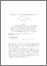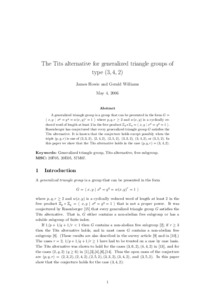# The Tits alternative for generalized triangle groups of type (3, 4, 2)

Howie, J and Williams, G (2008) 'The Tits alternative for generalized triangle groups of type (3, 4, 2).' Algebra and Discrete Mathematics, 2008 (4). 40 - 48.Preview
PDF
GeneralizedTriangleGps342.pdf

Download (154kB) | Preview

## Abstract

A generalized triangle group is a group that can be presented in the form G = h x, y | xp = yq = w(x, y)r = 1 i where p, q, r ? 2 and w(x, y) is a cyclically reduced word of length at least 2 in the free product Zp ? Zq = h x, y | xp = yq = 1i. Rosenberger has conjectured that every generalized triangle group G satisfies the Tits alternative. It is known that the conjecture holds except possibly when the triple (p, q, r) is one of (2, 3, 2), (2, 4, 2), (2, 5, 2), (3, 3, 2), (3, 4, 2), or (3, 5, 2). Building on a result of Benyash-Krivets and Barkovich from this journal, we show that the Tits alternative holds in the case (p, q, r) = (3, 4, 2).

Item Type: Article Q Science > QA Mathematics Faculty of Science and Health > Mathematical Sciences, Department of Jim Jamieson 04 Jan 2012 12:26 18 Oct 2017 16:18 http://repository.essex.ac.uk/id/eprint/1798

### Actions (login required)View Item